How to Add Fractions with Unlike Denominators - The Habitat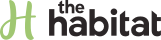Home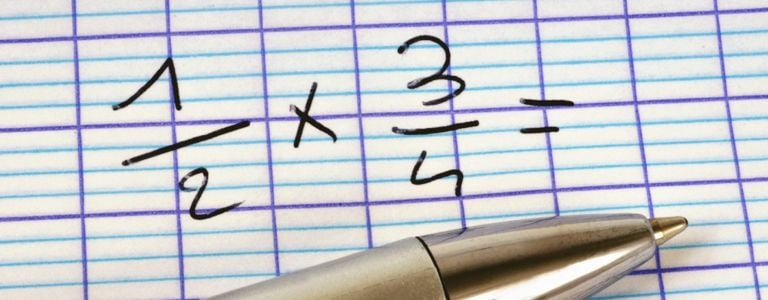A lot of people have trouble solving math problems. If you are one of them instead of struggling, you should simply look for online tutorials that can help you figure out problems. Fractions are one mathematical challenge that a lot of people struggle with, especially when there are different denominators. The good news is even with the bottom numbers are different, you can get an answer without breaking a sweat. The following is a quick guide to help you add fractions like a pro, with or without similar denominators so you can breeze through your work.

01

## Write Out the Fractions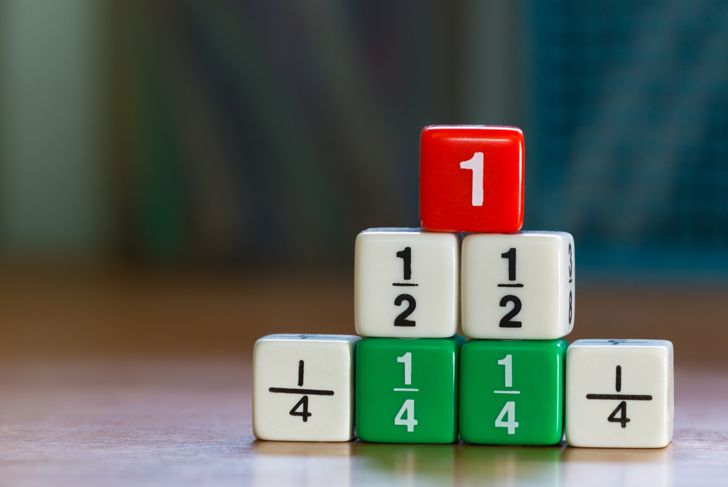First off, find yourself a pen and some paper and write down the fractions next to each other. Trying to do math in your head can make a simple task even more difficult, especially when the fractions have different denominators. You want to write them next to each other so you can clearly see what you are comparing. The example below will be completed as we work through the steps so you can see how the final answer comes to be.

Example: 1/3 + 3/4

02

## Find the Common DenominatorYou cannot add fractions that have different denominators until you identify a common denominator that they can share. The best way to do this is to find a multiple that they both share. If the numbers are small, simply multiply them against each other to find out what the common denominator is going to be.

Example: 3 x 4 = 12. This means that we will use 12 as the common denominator for our equation.

03

## Multiple Each of the first numbers by the Bottom Number of the Second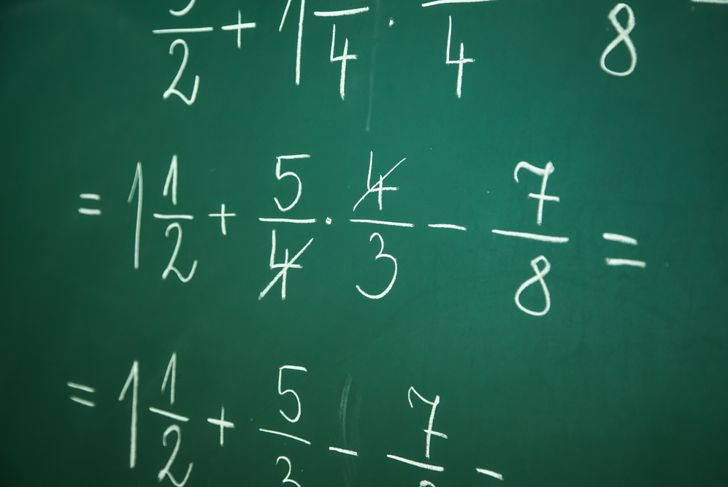This sounds confusing, but it isn’t once you get down to the equation. You want to get both fractions to have the bottom denominator of 12, so you do this by multiplying by the bottom number of the other. So, for instance, you will multiply 1/3 by 4/4, and then you will multiply 3/4 by 3/3. It seems like you are changing the numbers, but in reality, 3/3 or 4/4 is the same as multiplying by 1, and any number multiplied by one is the same. It is the same fraction, but we are just changing the way it looks to make the math easier.

Example: 1/3 x 4/4 = 4/12

04

## Multiple Both Numbers of the Second Fraction by the Bottom Number of the First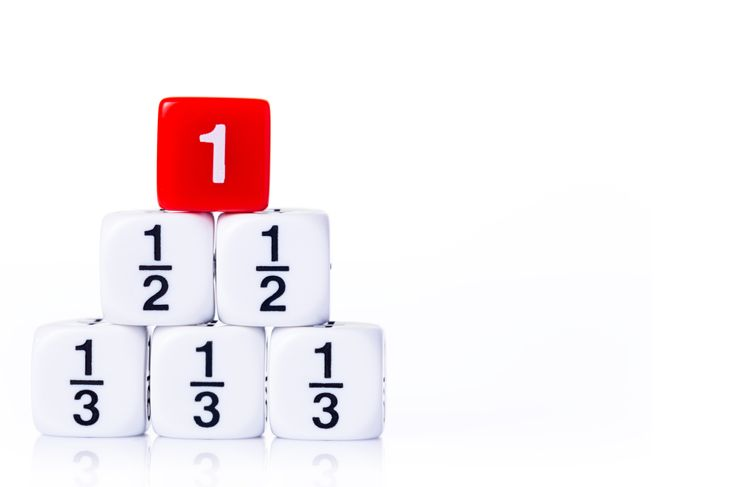This is the exact same thing we just did, only this time we are changing the fraction of the second number in the equation. Once again, we are really just multiplying by one, so the number of the fraction is not changing at all.

Example: 3/4 x 3/3 = 9/12

05

## Write out the New Fractions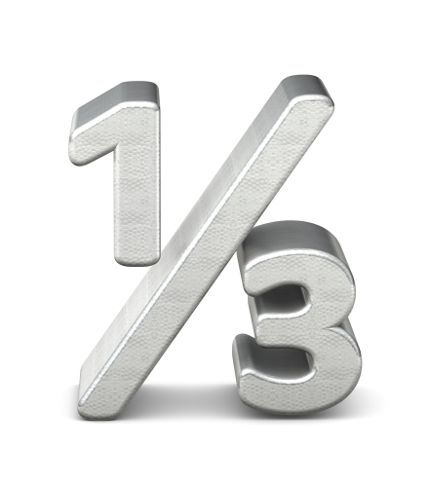Now that they both have the same denominator, we want to line the fractions up next to each other to make the math easier. By placing them side by side we get a cleaner image, and now that the numbers are the same the answer will seem a lot closer.

Example: Our new equation is 4/12 + 9/12

06Start the problem by looking at the numerators (the top numbers of the fraction). Then add the two numbers together and carry them into the solution. This will be the numerator of our answer.

Example: 4+9 = 13. That makes our new numerator 13.

07

## Carry the Common Denominator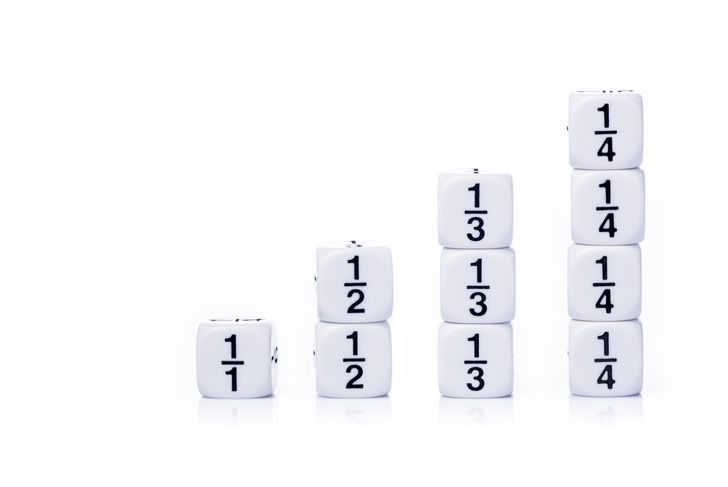When you add fractions, you only add the top number. That means that the common denominator will not change once you add the two fractions together. Since our common denominator was 12, it will remain our denominator in the solution.

08

## Put the Numbers Together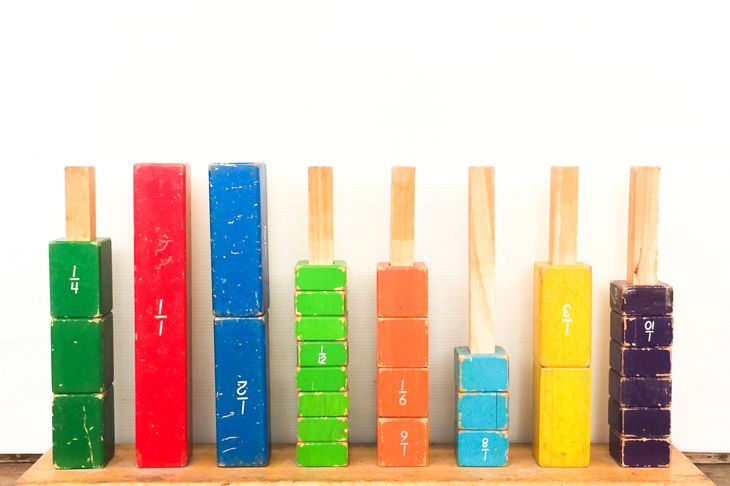Now that we know the denominator and the numerator, we can put them together. Remember, the numerator goes on top, and the denominator goes on the bottom. Therefore, we now have the solution to our math problem.

Example: 13/12

09

## Simplify and ReduceAfter you find the solution, you need to simplify or reduce your fraction. Our answer is a prime number so it cannot be simplified, but if we had a result like 6/8 then we would divide by two, and the final answer would be 3/4. However, our answer can be reduced, because the numerator is higher than the denominator.

Example: 13/12 reduces to 1 1/12

10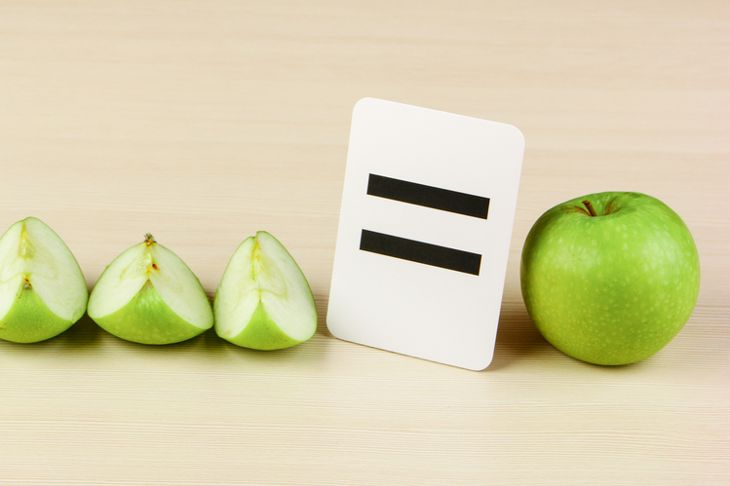Now that you know how to solve equations that ask you to add fractions with different denominators try a few examples on your own as practice. The more you complete these types of problems, the easier it will be to solve fraction problems in the future. This can help you out while measuring anything in the future from foods to medicines to chemicals in the lab. Here are some practice equations to get you started.

Example: 1/3 + 1/2

1/4 + 2/5

3/4 + 1/2